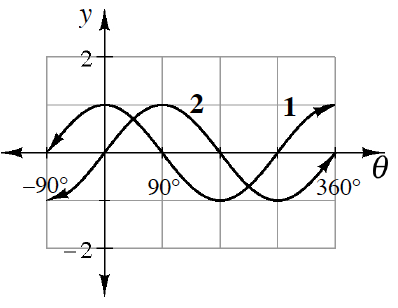### Home > CCA2 > Chapter Ch7 > Lesson 7.1.4 > Problem7-65

7-65.Daniel sketched the graphs at right for $y = \sinθ$ and $y = \cosθ$.

Unfortunately, he forgot to label the graphs, and now he cannot remember which graph goes with which equation. Explain to Daniel how he can tell (and remember!) which graph is $y = \sinθ$ and which is $y = \cosθ$.

Look at the $y$-intercepts. What is $\cos\left(0^\circ\right)$? $\sin\left(0^\circ\right)$?